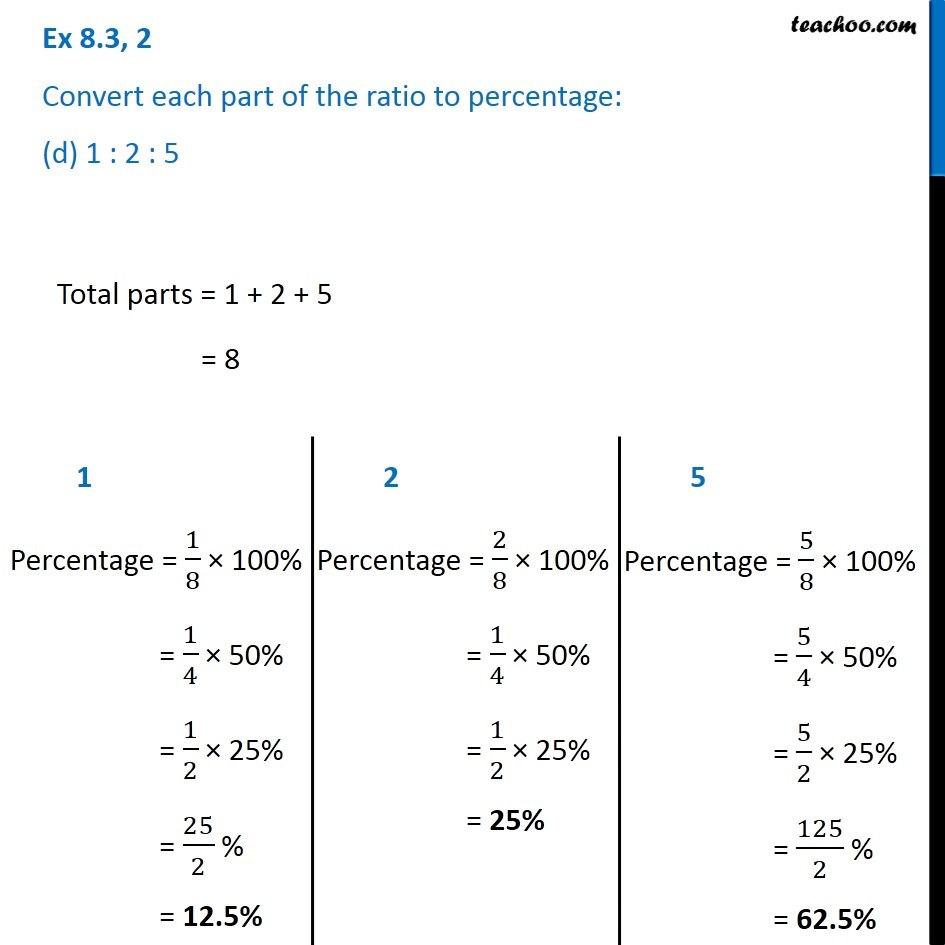Ex 8.3

Chapter 8 Class 7 Comparing Quantities
Serial order wiseGet live Maths 1-on-1 Classs - Class 6 to 12

### Transcript

Ex 8.3, 2 Convert each part of the ratio to percentage: (d) 1 : 2 : 5 Total parts = 1 + 2 + 5 = 8 1 Percentage = 1/8 × 100% = 1/4 × 50% = 1/2 × 25% = 25/2 % = 12.5% 2 Percentage = 2/8 × 100% = 1/4 × 50% = 1/2 × 25% = 25% 5 Percentage = 5/8 × 100% = 5/4 × 50% = 5/2 × 25% = 125/2 % = 62.5%